# QlikView App Dev

Discussion Board for collaboration related to QlikView App Development.

Announcements
QlikWorld 2023, a live, in-person thrill ride. Save \$300 before February 6: REGISTER NOW!
cancel
Showing results for
Did you mean: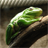Creator

Hi all,

I'm trying to show in a table the number of new buyers we have to each branch. New Buyers are buyers that have never bought before.

 count(

 {< UNIT.STOCKSTATUS = {'Sold'}, BUYER.B_NO = p({=\$(=\$(vRptYrMthSeqTY)-0)"}>}) * e({=\$(=\$(vRptYrMthSeqTY)-11)"}>}) * e({=\$(=\$(vRptYrMthSeqTY)-3)"}>}), BUYER.B_CATEGORY = {'*'}-{'PR'}

Could someone check to see if my set analysis is correct please?

Thanks

1 Solution

Accepted SolutionsCreator
Author

Hi all,

Thanks for everyone's responses I have managed to solve this now.

 count(

 {< UNIT.STOCKSTATUS = {'Sold'},BUYER.B_CATEGORY = {'*'}-{'PR'},VENDOR.C_GROUP = {'TCG'},SOLD.YrMthSeq = {"<=\$(=\$(vRptYrMthSeqTY)-0) >=\$(=\$(vRptYrMthSeqTY)-0)"}, BUYER.B_NO = e({=\$(=\$(vRptYrMthSeqTY)-11)"},BUYER.B_CATEGORY = {'*'}-{'PR'},UNIT.STOCKSTATUS = {'Sold'},VENDOR.C_GROUP = {'TCG'}>})

Thanks

9 Replies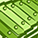Champion

Try like below one,

count({<

UNIT.STOCKSTATUS = {'Sold'},

SOLD.YrMthSeq =  {'<=\$(vRptYrMthSeqTY))'},

What is the value of the variable vRptYrMthSeqTY.? Also can you explain below statement!!

p({<SOLD.YrMthSeq =  {"<=\$(=\$(vRptYrMthSeqTY)-0)  >=\$(=\$(vRptYrMthSeqTY)-0)"}>}) *

e({<SOLD.YrMthSeq =  {"<=\$(=\$(vRptYrMthSeqTY)-4)  >=\$(=\$(vRptYrMthSeqTY)-11)"}>}) *

e({<SOLD.YrMthSeq =  {"<=\$(=\$(vRptYrMthSeqTY)-1)  >=\$(=\$(vRptYrMthSeqTY)-3)"}>}),

It would be helpful to guide you.Creator
Author

SOLD.YrMthSeq =  {"<=\$(=\$(vRptYrMthSeqTY)-0)  >=\$(=\$(vRptYrMthSeqTY)-0)"}>})

The above is a calendar we use -0 is September so -1 would be August -2 July and so on.

p({<SOLD.YrMthSeq =  {"<=\$(=\$(vRptYrMthSeqTY)-0)  >=\$(=\$(vRptYrMthSeqTY)-0)"}>}) *

e({<SOLD.YrMthSeq =  {"<=\$(=\$(vRptYrMthSeqTY)-4)  >=\$(=\$(vRptYrMthSeqTY)-11)"}>}) *

e({<SOLD.YrMthSeq =  {"<=\$(=\$(vRptYrMthSeqTY)-1)  >=\$(=\$(vRptYrMthSeqTY)-3)"}>}),

With the above set analysis I am trying to say the buyer who bought in September but exclude the ones that bought 11 months before September.

I could write this as e({<SOLD.YrMthSeq =  {"<=\$(=\$(vRptYrMthSeqTY)-1)  >=\$(=\$(vRptYrMthSeqTY)-11)"}>})

I am very new to e's and p's by the way so I could be completely wrong!!Champion

Hi Gareth,

Ok. I just want to know the value of variable \$(vRptYrMthSeqTY).? I guess we don't need P() and E(). Simply we can mention the month numbers.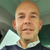Creator

For a better performance, solve this in your script! See New/Lost/Returning/Loyal Customers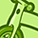Creator III

Hi,

You may try the below expression:

count(

{<UNIT.STOCKSTATUS = {'Sold'},

BUYER.B_CATEGORY = {'*'}-{'PR'} , SOLD.YrMthSeq>} Sales_Amount)<=0"}

Idea is to find out the buyers having zero sales before September (or selected month) along with other sets. Considered that Sales_Amount is the field containing brought value by Buyer.

Regards,

SomCreator
Author

Hi Tamil,

The value is a number so \$(vRptYrMthSeqTY). would equal 57 its the number of the month we are in.

Are you thinking about something like this,

 count({

SOLD.YrMthSeq = {"<=\$(=\$(vRptYrMthSeqTY)-0) >=\$(=\$(vRptYrMthSeqTY)-0)"}>

-

<UNIT.STOCKSTATUS = {'Sold'},  BUYER.B_CATEGORY -= {'PR'},

SOLD.YrMthSeq = {"<=\$(=\$(vRptYrMthSeqTY)-1) >=\$(=\$(vRptYrMthSeqTY)-11)"}

>}Champion

Hi Gareth,

Sorry for the late reply. Yes, i thought the same. Any issues in the above set analysis which you mentioned.

Edit: Did you alter your first expression like this and try.

 count(
{<
UNIT.STOCKSTATUS = {'Sold'},
 >}Creator
Author

Hi all,

Thanks for everyone's responses I have managed to solve this now.

 count(

 {< UNIT.STOCKSTATUS = {'Sold'},BUYER.B_CATEGORY = {'*'}-{'PR'},VENDOR.C_GROUP = {'TCG'},SOLD.YrMthSeq = {"<=\$(=\$(vRptYrMthSeqTY)-0) >=\$(=\$(vRptYrMthSeqTY)-0)"}, BUYER.B_NO = e({=\$(=\$(vRptYrMthSeqTY)-11)"},BUYER.B_CATEGORY = {'*'}-{'PR'},UNIT.STOCKSTATUS = {'Sold'},VENDOR.C_GROUP = {'TCG'}>})Glad that you solved it.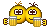.